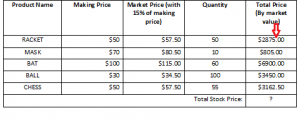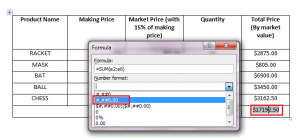# How to use formula in word table

We know that Excel is one of the popular applications to do different types of numerical calculation. But if you are not familiar with Excel or don’t like to use Excel and want to do some simple mathematical calculation on table data in word then it is possible to do. You don’t need to learn or use Excel to do the task.  Simple numerical calculation can be easily performed by using formula option of word.  You can do calculation by using many common formula functions and get your desired outputs from the table data. This article will help you to learn how you can use formula on tabular data in word and make numeric calculation easier.

Suppose, the following table data is given and you need to calculate market price based on making price,  total price based on quantity and total stock price using formula.First of all, we need to use a formula to calculate market price of the product based on making price.  Select the table and click on Layout tab. Formula option will be found here.Select the cell where you want to add formula and Click formula option to open formula dialog box. To calculate market price by adding 15% of making price, the formula for cell b2 will be “=b2+(b2*15/100)”.Type this formula in dialog box to calculate the price for RACKET.To calculate the market price of other products, you need to write the same formula for other cells. In Excel sheet, it can be done very easily by dragging cell. But this feature is not available in word. To make the task easier, press Alt + F9 to open the formula in the cell. Copy and paste the formula on all required cells where you want to use this formula. Now edit the formula based on cell location.Now, again press Alt+F9 to hide the formula. Select all cells where formula are used and press F9. Do right align for all price data. Now you will see that the market price of all products is calculated properly.The formula of total price will be “=(c2*d2)” for cell e2. You can copy and paste the formula for total price field by following above steps.The table will be looked like the following image after edit.Now, you have to write the formula for total stock price based on market value. To do this, select the cell and open formula dialog box. To add all values from cell e2 to cell e6, the formula is “=sum(e2:e6)” . Type this formula in formula text box and click OK button.After adding the formula, the total stock price will be shown.There is option in formula dialog box to format the numeric value. If you want to format the total stock price then select the cell value and open the formula dialog box. Select your desired format from Number format dropdown list. Here second option is selected to add comma after three digits.After format, the total stock price will be look like below.Paste function is another option of formula dialog box and you can do many useful mathematical and logical operations by using this. The use of this function will be explained in other articles.

You can show the full steps in the following video: